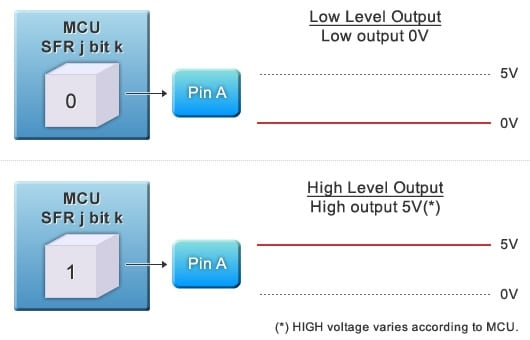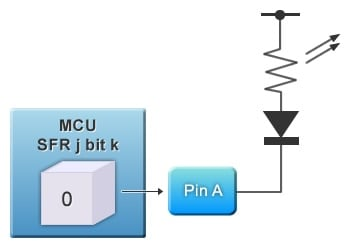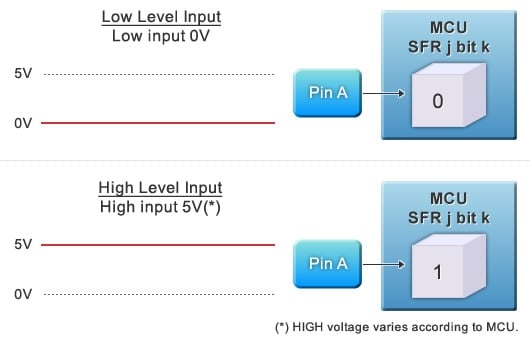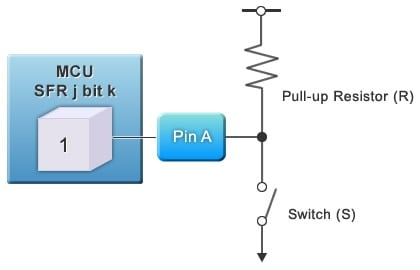We continue our review of MCU basics for aspiring embedded systems engineers. In earlier segments we covered MCU hardware, programming languages, and the development environment. This time we look at the basics of peripheral circuitry control.

## Special Function Registers (SFRs)

MCUs use a variety of internal registers to store values related to status and operations. Typical registers include the Program Counter, general-purpose registers, and SFRs. An MCU uses some of its SFRs special for the purpose of controlling peripheral circuitry. For example, it reads SFR values to obtain peripheral data such as count values, serial-port input, and general input. And it writes to SFRs as necessary to output data to peripherals and to control peripherals' settings and status.

## Control of External Peripheral Circuits

As an example, let’s look at how the MCU uses an SFR to handle output to and input from a specific peripheral.

1. The MCU writes 0 or 1 into a SFR bit to set output to the peripheral to LOW or HIGH level, which is connected to the SFR bit.
2. The MCU reads the value of a SFR bit to get the status from the connected peripheral.

In the Figures below, pin A is a general-purpose I/O line that connects to a specific bit (call it bit “k”) in one of the SFRs (call it SFR “j”).

Let’s first look at how the MCU uses the SFR bit to set the peripheral to either HIGH or LOW level.

• To set to LOW (0V), write 0 into bit k.
• To set to HIGH (5V), write 1 into bit k.Figure 1: General Purpose I/O Pin; Output Control

Assume, for example, that Pin A is connected to an LED, as shown in Figure 2. To turn the LED on, the MCU writes a 0 into SFR-j bit-k. To turn the LED off, it writes a 1 into the bit. This very simple design is actually used by many different types of peripherals. For example, the MCU can use the bit as a switch for turning a motor ON and OFF. (Since most MCUs cannot output enough current to drive a motor, the pin would typically connect to a drive circuit incorporating FETs or other transistors). More complex controls can be implemented by utilizing multiple I/O ports.Figure 2: LED On/Off Circuit Controlled by General I/O

Next, let’s see how the MCU uses the SFR bit to input the current status of the peripheral. All that is needed is to read the bit value.

• If the MCU reads 0 from SFR-j bit-k, it knows that the peripheral is inputting a LOW signal (0V) into Pin A.
• If the MCU reads 1 from SFR-j bit-k, it knows that the peripheral is inputting a HIGH signal (5V) into Pin A.Figure 3: General Purpose I/O Pin; Input Control

Figure 4 shows how an external switch can be set up so that MCU can read the switch setting through its SFR.

• When Switch S is OFF, voltage is pulled up by Resistor R, resulting into HIGH input into Pin A. This sets the value of the SFR bit (SFR-j bit-k) to 1.
• When Switch S is ON, the voltage into Pin A is LOW, and the SFR bit value resets to 0.

MCU can easily determine whether the switch is ON or OFF by reading the SFR bit.Figure 4: Implementing a Switch through General I/O

Each MCU incorporates numerous SFRs capable of implementing a wide range of functionalities. Programs can read these SFRs to get information about external conditions, and can write these SFRs to control external behavior. If an MCU were a human being, its SFRs would be its hands, its feet, and its five senses.

This concludes our introduction to peripheral control basics. In our next and final segment on MCU basics, we will be looking at interrupts. See you then.

In the concluding session of this MCU Introduction series, we will be looking at interrupts. Interrupt processing is one of the most important features of MCU programming.